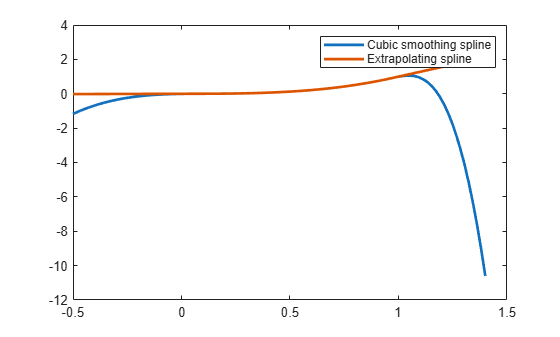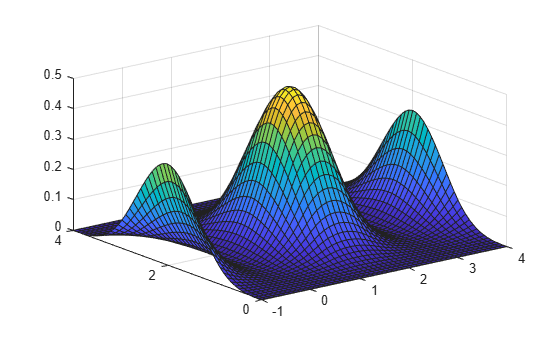Documentation

# fnxtr

Extrapolate spline

## Syntax

``g = fnxtr(f,order)``
``g = fnxtr(f)``

## Description

example

````g = fnxtr(f,order)` returns a spline of order `order` that extrapolates the spline `f`. `g` equals `f` on its basic interval, but `g` is a polynomial of the given order outside that interval. `g` satisfies at least `order` smoothness conditions at the ends of the basic interval of `f`, that is, at the new breaks. It is most useful to use a positive value of `order` that is smaller than the order of `f`.```
````g = fnxtr(f)` uses an extrapolation order equal to 2. It is equivalent to `fnxtr(f,2)`.```

## Examples

collapse all

Create a cubic smoothing spline on the unit interval.

```x = rand(1,21); spline = csaps(x,x.^3); ```

Create an extrapolating spline of order 2.

```order = 2; extrSpline = fnxtr(spline,order);```

Plot the original spline together with the extrapolating spline.

```fnplt(spline,[-.5 1.4]) hold on fnplt(extrSpline,[-.5 1.4]) legend('Cubic smoothing spline','Extrapolating spline') hold off```Create and plot a bivariate B-spline.

```spline = spmak({0:3,0:4},1); fnplt(spline)```Create an extrapolating spline. To extrapolate in the first variable only, specify a negative integer as the extrapolation order in the second variable.

```order = [3,-1]; extrSpline = fnxtr(spline,order); fnplt(extrSpline)```## Input Arguments

collapse all

Spline to extrapolate, specified as a structure. `f` must be a spline in B-form, BBform, or ppform.

Data Types: `struct`

Order of extrapolating spline, specified as an integer or a vector of integers.

If `order` is zero, then the extrapolating spline describes the same spline as `fn2fm(f,'B-')`, but is in ppform and has a larger basic interval.

If `order` is at least as large as the order of `f`, then the extrapolating spline is the same spline as `gn2fm(f,'pp')`, but uses two more pieces and has a larger basic interval.

If `f` is m-variate, then `order` can be a vector with m elements, where `order(i)` is the order used to extrapolate in the `i`-th variable. To exclude the `i`-th variable from being used in the extrapolation, specify `order(i)` as a negative integer.

Example: 1

## Output Arguments

collapse all

Spline in ppform, returned as a structure with these fields.

Form of the spline, returned as `pp`. `pp` indicates that the spline is given in piecewise polynomial form.

Knot positions of the spline, returned as a vector or as a cell array of vectors for multivariate data. Vectors contain strictly increasing elements that represent the start and end of each of the intervals over which the polynomial pieces are defined.

Coefficients of polynomials for each piece, returned as a matrix or as an array for multivariate data.

Number of polynomial pieces describing the spline, returned as a scalar or as a vector of numbers of pieces in each variable for multivariate data.

Order of the polynomial function describing each polynomial piece of the spline, returned as a scalar or as a vector containing the order in each variable for multivariate data.

Dimensionality of the target function, returned as a scalar.

## See Also

#### Machine Learning Challenges: Choosing the Best Classification Model and Avoiding Overfitting

Download white paper